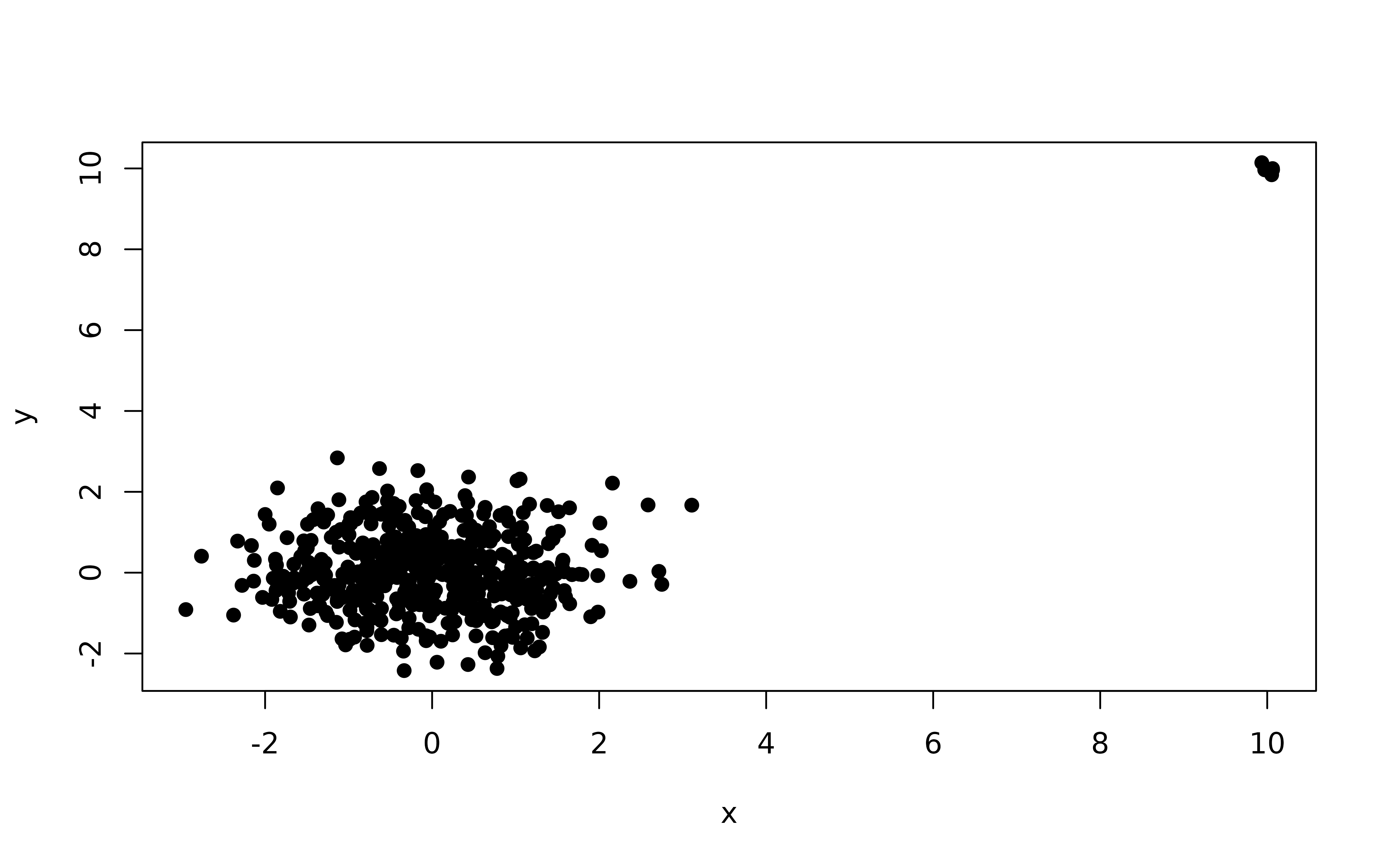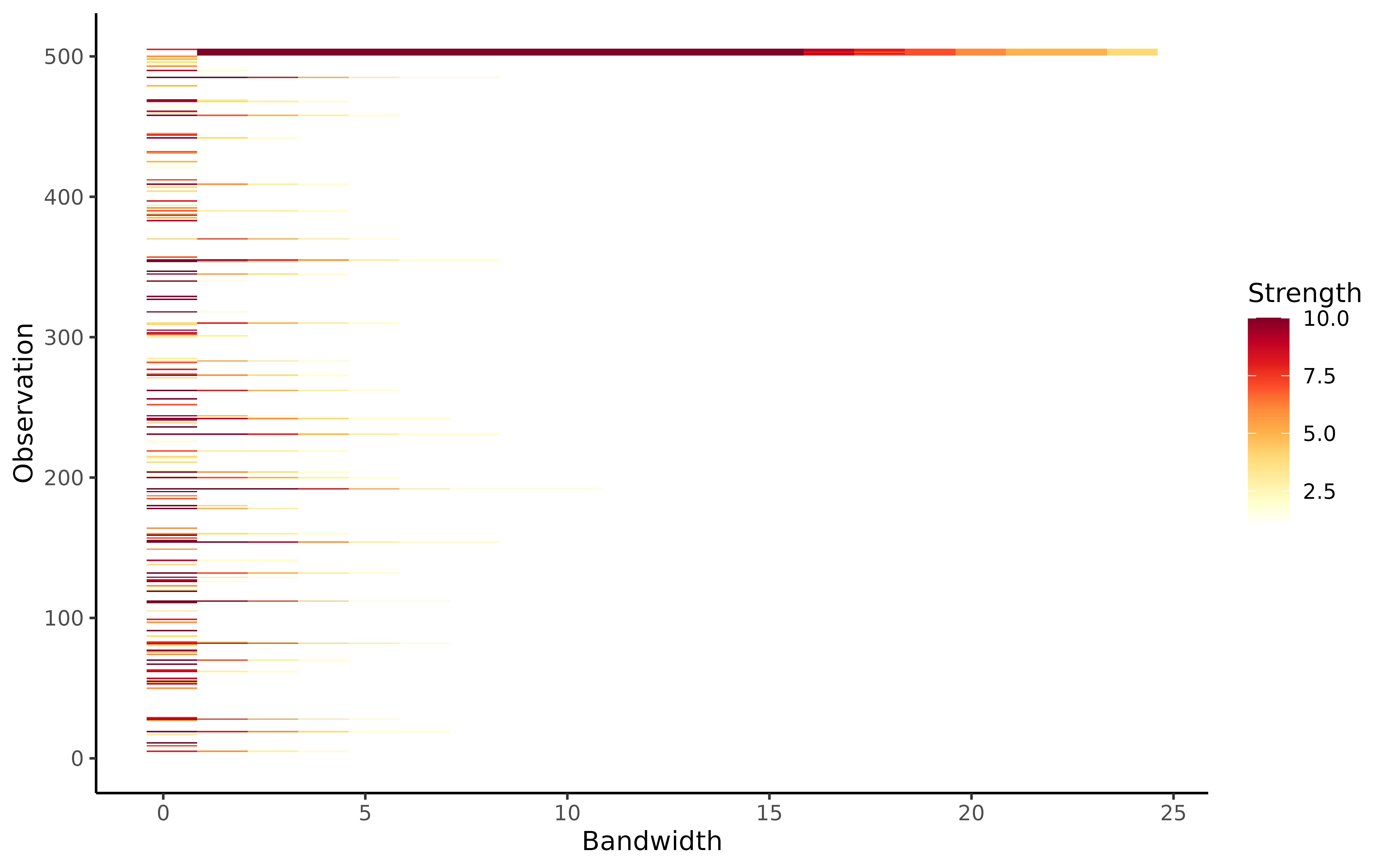This function plots outlier persistence for a range of significance levels using the algorithm lookout, an outlier detection method that uses leave-one-out kernel density estimates and generalized Pareto distributions to find outliers.

# S3 method for persistingoutliers
autoplot(object, alpha = object\$alpha, ...)

## Arguments

object The output of the function persisting_outliers. The significance levels to plot. Other arguments currently ignored.

A ggplot object.

## Examples

X <- rbind(
data.frame(
x = rnorm(500),
y = rnorm(500)
),
data.frame(
x = rnorm(5, mean = 10, sd = 0.2),
y = rnorm(5, mean = 10, sd = 0.2)
)
)
plot(X, pch = 19)outliers <- persisting_outliers(X, unitize = FALSE)
autoplot(outliers)